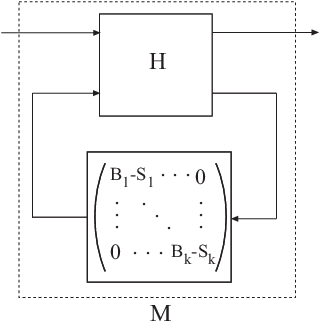Main Content

# getLFTModel

Decompose generalized LTI model

## Syntax

```[H,B,S] = getLFTModel(M) ```

## Description

`[H,B,S] = getLFTModel(M)` extracts the components `H`, `B`, and `S` that make up the Generalized matrix or Generalized LTI model `M`. The model `M` decomposes into `H`, `B`, and `S`. These components are related to `M` as shown in the following illustration.The cell array `B` contains the Control Design Blocks of `M`. The component `H` is a numeric matrix, `ss` model, or `frd` model that describes the fixed portion of `M` and the interconnections between the blocks of `B`. The matrix `S` `= blkdiag(S1,...,Sk)` contains numerical offsets that ensure that the interconnection is well-defined when the current (nominal) value of `M` is finite.

You can recombine `H`, `B`, and `S` into `M` using `lft`, as follows:

`M = lft(H,blkdiag(B{:})-S);`

## Input Arguments

 `M` Generalized LTI model (`genss` or `genfrd`) or Generalized matrix (`genmat`).

## Output Arguments

 `H` Matrix, `ss` model, or `frd` model describing the numeric portion of `M` and how it the numeric portion is connected to the Control Design Blocks of `M`. `B` Cell array of Control Design Blocks (for example, `realp` or `tunableSS`) of `M`. `S` Matrix of offset values. The software might introduce offsets when you build a Generalized model to ensure that `H` is finite when the current (nominal) value of `M` is finite.

## Tips

• `getLFTModel` gives you access to the internal representation of Generalized LTI models and Generalized Matrices. For more information about this representation, see Internal Structure of Generalized Models.

## See Also

Introduced in R2011a

## Support

#### Learn how to automatically tune PID controller gains

Download code examples